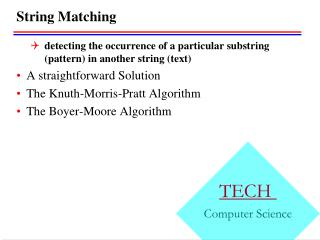DownloadDownload PresentationString Matching

# String Matching

Télécharger la présentation## String Matching

- - - - - - - - - - - - - - - - - - - - - - - - - - - E N D - - - - - - - - - - - - - - - - - - - - - - - - - - -
##### Presentation Transcript

1. String Matching detecting the occurrence of a particular substring (pattern) in another string (text) A straightforward Solution The Knuth-Morris-Pratt Algorithm The Boyer-Moore Algorithm

2. Straightforward solution • Algorithm: Simple string matching • Input: P and T, the pattern and text strings; m, the length of P. The pattern is assumed to be nonempty. • Output: The return value is the index in T where a copy of P begins, or -1 if no match for P is found.

3. int simpleScan(char[] P,char[] T,int m) • int match //value to return. • int i,j,k; • match = -1; • j=1;k=1; i=j; • while(endText(T,j)==false) • if( k>m ) • match = i; //match found. • break; • if(tj == pk) • j++; k++; • else • //Back up over matched characters. • int backup=k-1; • j = j-backup; • k = k-backup; • //Slide pattern forward,start over. • j++; i=j; • return match;

4. Analysis • Worst-case complexity is in (mn) • Need to back up. • Works quite well on average for natural language.

5. Finite Automata • Terminologies •  : the alphabet •  *: the set of all finite-length strings formed using characters from . • xy: concatenation of two strings x and y. • Prefix: a string w is a prefix of a string x if x=wy for some string y  *. • Suffix: a string w is a suffix of a string x if x= yw for some string y  *.

6. Finite Automata (cont’d)

7. Finite Automata, e.g.,

8. Algorithm

9. The Knuth-Morris-Pratt algorithm • Skip outer iteration I =3 • Skip first inner iteration testing “n” vs “n” at outer iteration i=4

10. Strategy • In general, if there is a partial match of j chars starting at i, then we know what is in position T[i]…T[i+j-1]. So we can save by • Skip outer iterations (for which no match possible) • Skip inner iterations (when no need to test know matches). • When a mismatch occurs, we want to slide P forward, but maintain the longest overlap of a prefix of P with a suffix of the part of the text that has matched the pattern so far. • KMP algorithm achieves linear time performance by capitalizing on the observation above, via building a simplified finite automaton: each node has only two links, success and fail.

11. Sliding the pattern for the KMP algorithm

12. The Knuth-Morris-Pratt Flowchart • Character labels are inside the nodes • Each node has two arrows out to other nodes: success link, or fail link • next character is read only after a success link • A special node, node 0, called “get next char” which read in next text character. • e.g. P = “ABABCB”

13. Construction of the KMP Flowchart • Definition:Fail links • We define fail[k] as the largest r (with r<k) such that p1,..pr-1 matches pk-r+1...pk-1.That is the (r-1) character prefix of P is identical to the one (r-1) character substring ending at index k-1. Thus the fail links are determined by repetition within P itself.

14. Algorithm: KMP flowchart construction • Input: P,a string of characters;m,the length of P. • Output: fail,the array of failure links,defined for indexes 1,...,m.The array is passed in and the algorithm fills it. • Step: • void kmpSetup(char[] P, int m, int[] fail) • int k,s • 1. fail=0; • 2. for(k=2;k<=m;k++) • 3. s=fail[k-1]; • 4. while(s>=1) • 5. if(ps==pk-1) • 6. break; • 7. s=fail[s]; • 8. fail[k]=s+1;

15. The Knuth-Morris-Pratt Scan Algorithm • int kmpScan(char[] P,char[] T,int m,int[] fail) • int match, j,k; • match= -1; • j=1; k=1; • while(endText(T,j)==false) • if(k>m) • match = j-m; • break; • if(k==0) • j++; k=1; • else if(tj==pk) • j++; k++; • else • //Follow fail arrow. • k=fail[k]; • //continue loop. • return match;

16. Analysis • KMP Flowchart Construction require 2m – 3 character comparisons in the worst case • The scan algorithm requires 2n character comparisons in the worst case • Overall: Worst case complexity is (n+m)

17. The Boyer-Moore Algorithm

18. Algorithm:Computing Jumps for the Boyer-Morre Algorithm • Input:Pattern string P:m the length of P;alphabet size alpha=|| • Output:Array charJump,defined on indexes 0,....,alpha-1.The array is passed in and the algorithm fills it. • void computeJumps(char[] P,int m,int alpha,int[] charJump) • char ch; int k; • for (ch=0;ch<alpha;ch++) • charJump[ch]=m; • for (k=1;k<=m;k++) • charJump[pk]=m-k;

19. Computing matchJump

20. Computing matchjump (e.g.,)

21. BoyerMooreScan Algorithm

22. Summary • Straightforward algorithm: O(nm) • Finite-automata algorithm: O(n) • KMP algorithm: O(n+m) • Relatively easier to implement • Do not require random access to the text • BM algorithm: O(n+m), worst, sublinear average • Fewer character comparison • The algorithm of choice in practice for string matcing